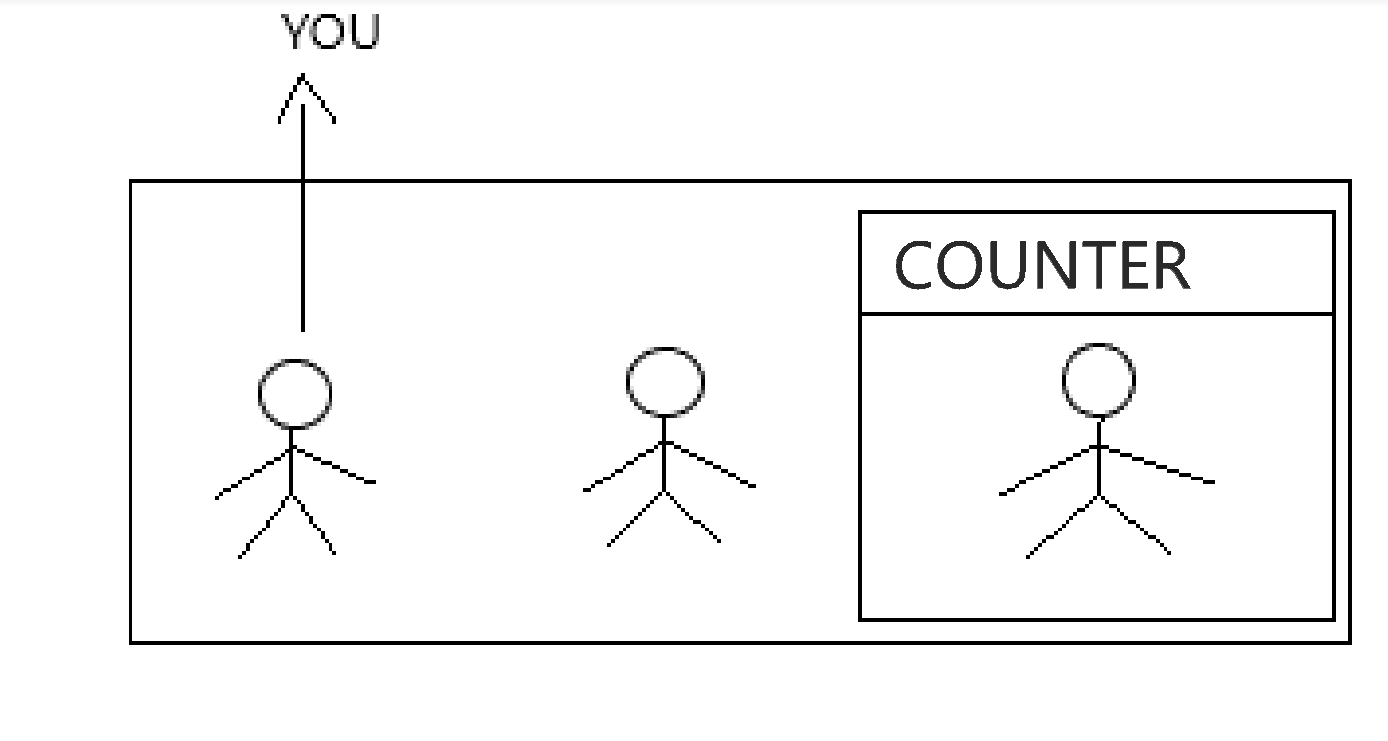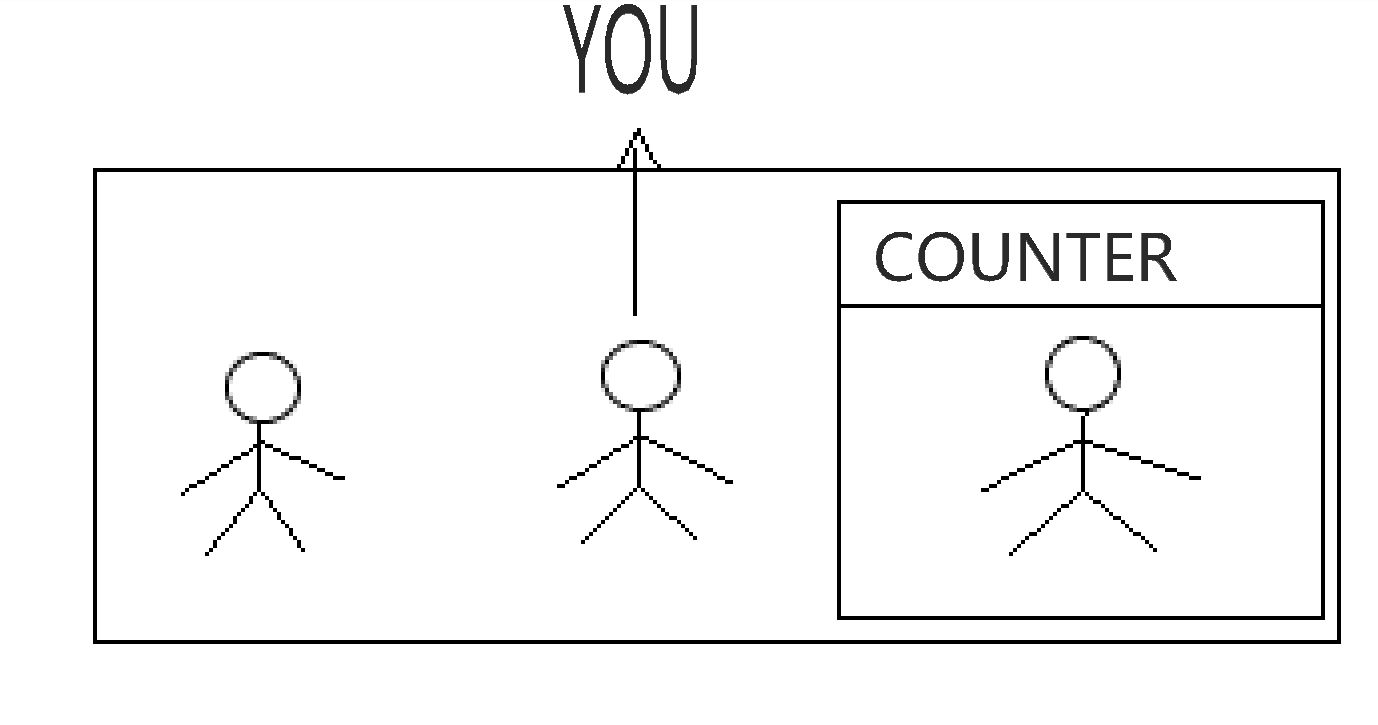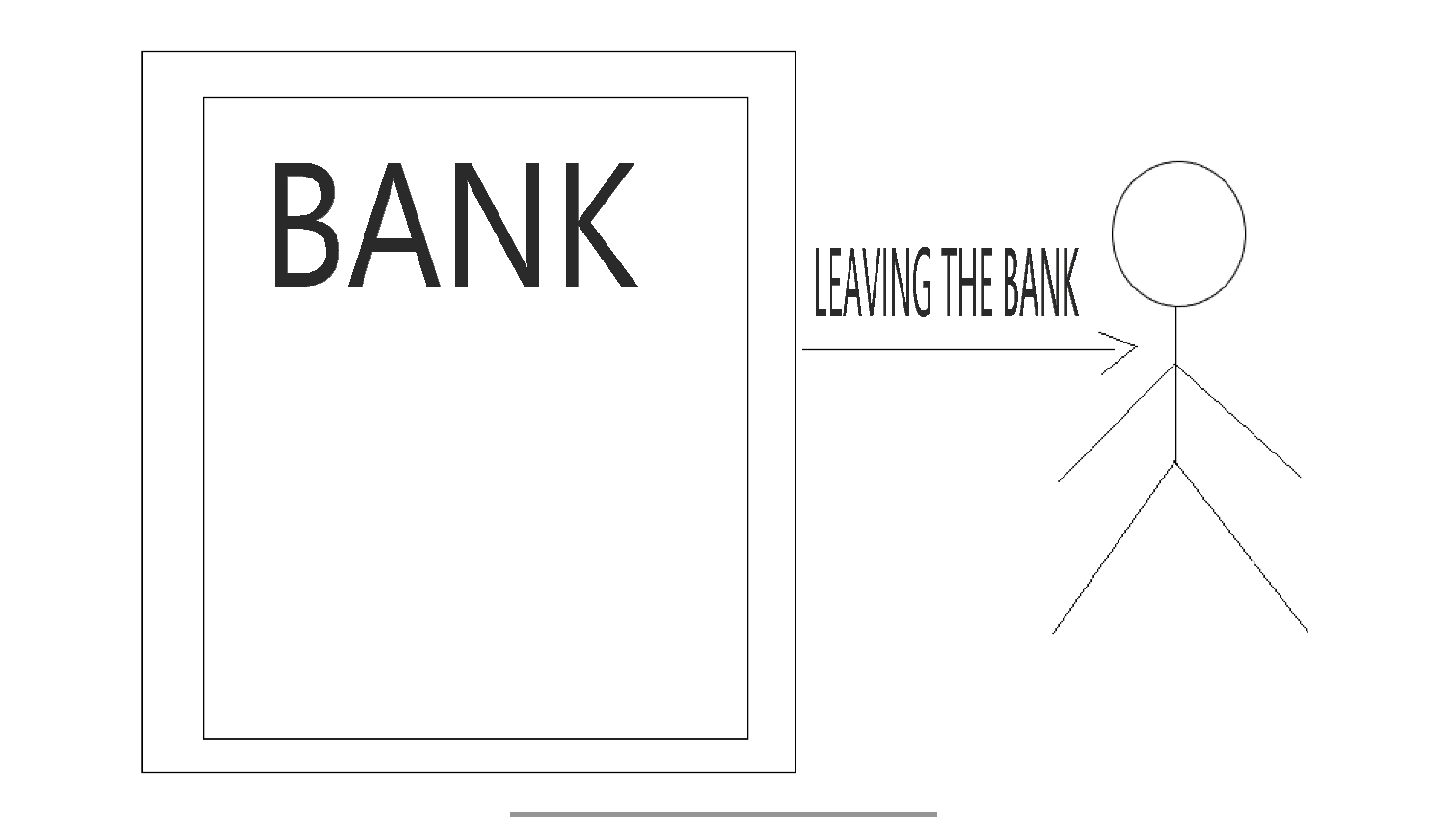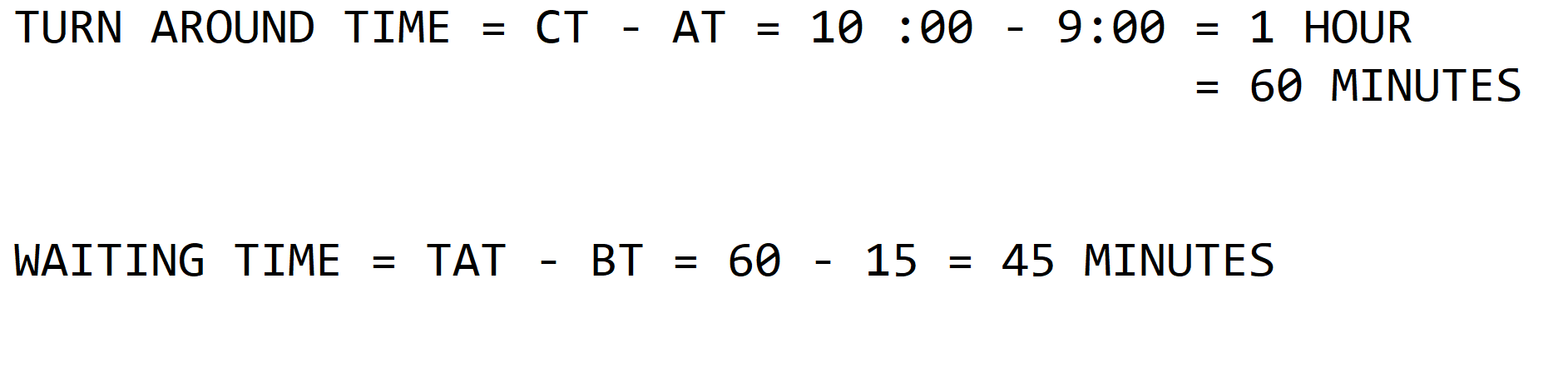×

Search anything:

# Terms in CPU Scheduling: Arrival, Burst, Turnaround, Completion Time and more

#### Software Engineering**Scheduling Metrics: Scheduling metrics use to measure something, there are different metrics.So let us start talking about 5 main types of Scheduling Metrics they are :-

Terms:

1. ARRIVAL TIME
2. WAITING TIME
3. BURST TIME
4. COMPLETION TIME
5. TURN AROUND TIME
6. Other terms: Response Time(RT), Throughput, Processor Utilization

We will take the example of bank and explain it in a detailed manner .

## 1) ARRIVAL TIME

Suppose you have reached the bank at 9 o'clock and this "point of time" is called as Arrival Time .

ARRIVAL TIME With Proper definition and Real World Example :-

Standard Definition :-
The "point of time " at which process enters the ready queue or state is called as ARRIVAL TIME and it is denoted as (AT).

Standard Definition :-
The "point of time " at which process enters the ready queue or state is called as ARRIVAL TIME and it is denoted as (AT).

Arrival Time (A.T.) = Completion Time (C.T.) - Turn Around Time (T.A.T)

Arrival Time (A.T.) = Completion Time (C.T.) - Turn Around Time (T.A.T)In the above diagram we can see that you are entering the bank at 9 0'clock and this "point of time" is called as ARRIVAL TIME ie (AT).

PROPERTIES:-

• Marks the entry point of the process in the queue.
• Computed before the execution of process.
• Related to the Ready State of the CPU.

## 2) WAITING TIME

And now you are waiting from 9 to 9:45 and this "duration" is called as Waiting Time.

WAITING TIME With Proper definition and Real World Example :-

Standard Definition:-
How much "duration" processes spend in the ready queue waiting their turn to get on the CPU is called as WAITING TIME and it is denoted as (WT).

Standard Definition:-
How much "duration" processes spend in the ready queue waiting their turn to get on the CPU is called as WAITING TIME and it is denoted as (WT).

Waiting time(WT) = Turnaround time(TAT) - Burst time(BT)

Waiting time(WT) = Turnaround time(TAT) - Burst time(BT)In this above diagram we can see that you are waiting from 9 to 9:45 and this "duration" is called as WAITING TIME ie (WT).

PROPERTIES:-

• The time process spent in the ready queue and for I/O completion.

• CPU Scheduling Algorithm doesn’t affect the amount of time during which a process executes or does I/O but only the amount of time that a process spends waiting in the ready queue.

• Waiting time has no such major effect.

## 3) Burst Time

Then after waiting a lot of time you have finally reached the counter and now from
9:45 to 10:00 your work at the counter has been done and this "duration" is called as the Burst Time.

Burst Time With Proper Definition and Real World Example :-

Standard Definition:-
How much "duration" required by the process to get execute on CPU is called as the BURST TIME and denoted as (BT).

Standard Definition:-
How much "duration" required by the process to get execute on CPU is called as the BURST TIME and denoted as (BT).

Burst Time (B.T.) = Completion Time (C.T.) - Waiting Time (W.T.)

Burst Time (B.T.) = Completion Time (C.T.) - Waiting Time (W.T.)In this above diagram we can see that after waiting a lot of time you have finally reached the counter and now from 9:45 to 10:00 your work at the counter has been done and this "duration" is called as the BURST TIME ie (BT).

PROPERTIES:-

• Marks the exit point of the process in the queue.

• Computed after the execution of process.

• Related to the Running State of the CPU.

## 4) Completion Time

Finally you are out of the bank and you are out at 10:00 and this "point of time" is called as Completion Time .

Completion Time With Proper Definition and Real World Example :-

Standard Definition :-
The "point of time " at which process complete its execution is called as COMPLETION TIME and it is denoted as (CT).

Standard Definition :-
The "point of time " at which process complete its execution is called as COMPLETION TIME and it is denoted as (CT).

Completion Time (C.T.)= Arrival Time (A.T.) + Turn Around Time (T.A.T)

Completion Time (C.T.)= Arrival Time (A.T.) + Turn Around Time (T.A.T)In this above diagram we can see that finally you are out of the bank and you are out at 10:00 and this "point of time" is called as COMPLETION TIME ie (CT).

Properties:-

• Completion time is the point of time at which a process completes its execution on the CPU and takes exit from the system.

• It is also called as exit time.

## 5)TURN AROUND TIMETAT = CT - AT

Where TAT = Turn Around Time
CT = Completion Time'
AT = Arrival Time

PROPERTIES:-

• The time since the process entered into ready queue for execution till the process completed it’s execution.

• Different CPU Scheduling algorithms produce different TAT for the same set of processes.

• The turnaround time is generally limited by the speed of the output device.

Difference between Turnaround Time and Waiting Time:

Turnaround Time PROPERTIES:-

• The time since the process entered into ready queue for execution till the process completed it’s execution.

• Different CPU Scheduling algorithms produce different TAT for the same set of processes.

• The turnaround time is generally limited by the speed of the output device.

Waiting Time PROPERTIES:-

• The time process spent in the ready queue and for I/O completion.

• CPU Scheduling Algorithm doesn’t affect the amount of time during which a process executes or does I/O but only the amount of time that a process spends waiting in the ready queue.

• Waiting time has no such major effect.

## Difference between Arrival Time and Burst Time:

Arrival Time PROPERTIES:-

• Marks the entry point of the process in the queue.

• Computed before the execution of process.

• Related to the Ready State of the CPU.

Burst Time PROPERTIES:-

• Marks the exit point of the process in the queue.

• Computed after the execution of process.

• Related to the Running State of the CPU.

POINTS TO REMEMBER ->

1. Arrival and Completion Time are the "Point of time" for example: 10 pm , 11 am etc.

2. Waiting Time , Burst Time and Turn around Time are nothing but the "Durations"
for example : duration of 1 hour , duration of 15 minutes etc.

There are other Scheduling Metrics they are :-

1. Response Time(RT): The time it takes to start responding to a request. It from first submission of the process until the first running.

RT = ( Time of first response ) - ( Time of submission of request )

1. Throughput: The number of processes that are completed per unit time.

Throughput = (Number of processes completed) / (Time unit)

1. Processor Utilization: The percentage of the time that CPU is busy, means not idle.

Processor utilization = (Processor busy time) / (Processor busy time + Processor idle time)

So this is all about the basic terminologies in CPU Scheduling in Simple Words with a real world example in this article at OpenGenus.